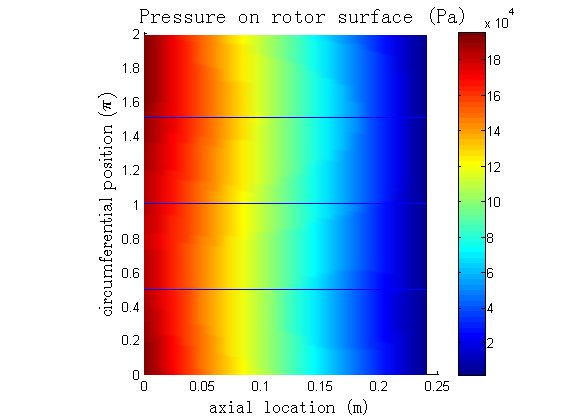Since I have spent a lot of time studying the flow inside the running clearances in pumps, it becomes attractive if the calculated values on a rotor surface could be drawn as contours. So I decided to write a piece of Matlab code to do the job for me. The simulation data is exported from CFX-Post.

In order to generate the contour, I utilize the patch function of Matlab. For patch to work you have to tell it the topological structure of the grid and geometry. Thus, you have to export geometry data from CFX-Post. The geometry data holds the coordinate of each node and the connections among nodes for each element face. You feed these information into patch together with the calculated values, such as pressure, on all the nodes. Then patch will give you the contour image.

A sample contour looks like this ↓:In fact, another Matlab function, griddata, seems more suitable for this purpose. I will look into that later.

The following list is the Matlab code. And associated m-files are listed here: